# Find Minimum, Maximum, and Average Value of PySpark Dataframe column

• Last Updated : 29 Jun, 2021

In this article, we are going to find the Maximum, Minimum, and Average of particular column in PySpark dataframe.

For this, we will use agg() function. This function Compute aggregates and returns the result as DataFrame.

Syntax: dataframe.agg({‘column_name’: ‘avg/’max/min})

Where,

• dataframe is the input dataframe
• column_name is the column in the dataframe

Creating DataFrame for demonstration:

## Python3

 `# importing module``import` `pyspark`` ` `# importing sparksession from pyspark.sql ``# module``from` `pyspark.sql ``import` `SparkSession`` ` `# creating sparksession and giving an app``# name``spark ``=` `SparkSession.builder.appName(``'sparkdf'``).getOrCreate()`` ` `# list  of students  data``data ``=` `[[``"1"``, ``"sravan"``, ``"vignan"``, ``67``, ``89``],``        ``[``"2"``, ``"ojaswi"``, ``"vvit"``, ``78``, ``89``],``        ``[``"3"``, ``"rohith"``, ``"vvit"``, ``100``, ``80``],``        ``[``"4"``, ``"sridevi"``, ``"vignan"``, ``78``, ``80``],``        ``[``"1"``, ``"sravan"``, ``"vignan"``, ``89``, ``98``],``        ``[``"5"``, ``"gnanesh"``, ``"iit"``, ``94``, ``98``]]`` ` `# specify column names``columns ``=` `[``'student ID'``, ``'student NAME'``,``           ``'college'``, ``'subject 1'``, ``'subject 2'``]`` ` `# creating a dataframe from the lists of data``dataframe ``=` `spark.createDataFrame(data, columns)`` ` `# display dataframe``dataframe.show()`

Output: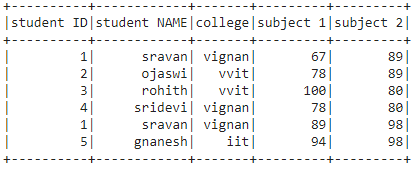### Finding Average

Example 1: Python program to find the average of dataframe column

## Python3

 `# find average of subjects column``dataframe.agg({``'subject 1'``: ``'avg'``}).show()`

Output: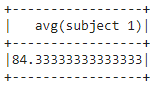Example 2: Get average from multiple columns

## Python3

 `# find average of multiple  column``dataframe.agg({``'subject 1'``: ``'avg'``,``               ``'student ID'``: ``'avg'``,``               ``'subject 2'``: ``'avg'``}).show()`

Output: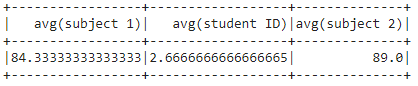### Finding Minimum

Example 1: Python program to find the minimum value in dataframe column.

## Python3

 `# minimum value from student ID column``dataframe.agg({``'student ID'``: ``'min'``}).show()`

Output: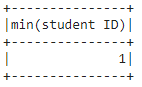Example 2: Get minimum value from multiple columns

## Python3

 `# minimum value from multiple column``dataframe.agg({``'college'``: ``'min'``,``               ``'student NAME'``: ``'min'``,``               ``'student ID'``:``'min'``}).show()`

Output: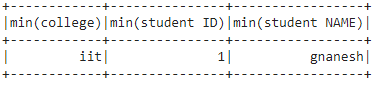### Finding Maximum

Example 1: Python program to find the maximum value in dataframe column

## Python3

 `# maximum value from student ID column``dataframe.agg({``'student ID'``: ``'max'``}).show()`

Output: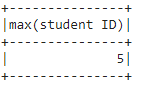Example 2: Get maximum value from multiple columns

## Python3

 `# maximum value from multiple column``dataframe.agg({``'college'``: ``'max'``,``               ``'student NAME'``: ``'max'``,``               ``'student ID'``:``'max'``}).show()`

Output: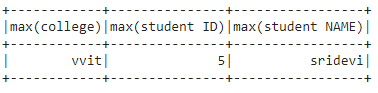My Personal Notes arrow_drop_up